Get instant live expert help with Excel or Google Sheets“My Excelchat expert helped me in less than 20 minutes, saving me what would have been 5 hours of work!”

#### Post your problem and you’ll get Expert help in seconds.

Your message must be at least 40 characters
Our professional Expert are available now. Your privacy is guaranteed.

# How to add up on excel

Adding numbers or cells can be done by simple addition or by using the functions SUM and SUBTOTAL.   This article assists all levels of Excel users on how to add up.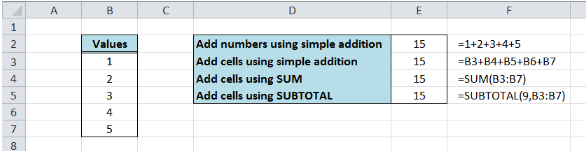Figure  1. Final result:  How to add up

## How to add numbers in a single cell

Using simple addition, we can add numbers by manually entering the numbers and using the addition “plus” symbol.

Formula:

`=1+2+3+4+5`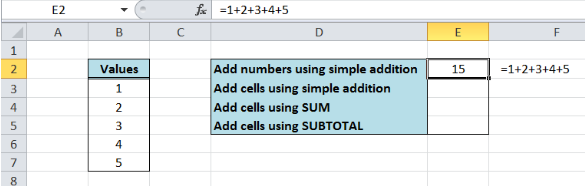Figure 2.  Add numbers using simple addition

We can add cells by simple addition of the cell references or by the use of the functions SUM and SUBTOTAL.

We can use simple addition to add cells by manually entering the cell references and inserting addition “plus” symbols in between the values.

### Formula

`=B3+B4+B5+B6+B7`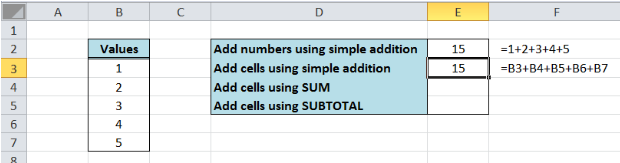Figure 3. Add cells using simple addition

The simple addition method is easy to use even for beginners but it becomes tedious and prone to errors when applied to a large set of cells.  A simpler method in how to add cells can be done by using the SUM function.

## How to add up using SUM function?

The SUM function makes it easier and faster to sum a large data set and it can add up to 255 values.

### Formula

`=SUM(B3:B7)`

The argument in the SUM function is the range of cells that we want to sum, B3:B7.  Instead of entering the cell references one by one like in simple addition, SUM allows us to add cells quickly using range of cells or arrays.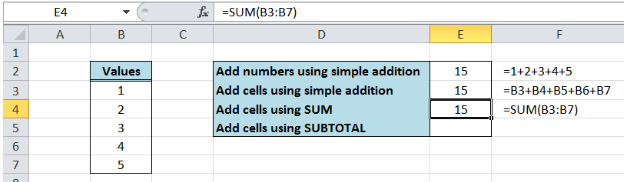Figure 4.  Add cells using the SUM function

## How to add up using SUBTOTAL function?

SUBTOTAL is another Math/Trig function that returns the subtotal of selected cells.  It lets us choose from a set of functions and SUM is one of them.

### Formula

`=SUBTOTAL(9,B3:B7)`

The first argument in the SUBTOTAL function is “9”, which is the function number of SUM.  Below is the list of functions that we can use within SUBTOTAL: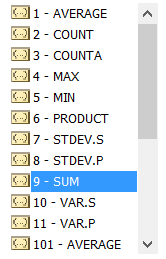Figure 5.  Function options in SUBTOTAL

The second argument is the range of cells that we want to sum B3:B7.  The result is 15, which is the sum of the numbers 1, 2, 3, 4 and 5.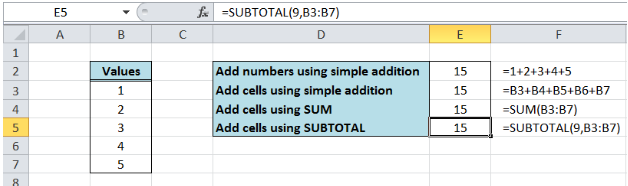Figure 6.  Add cells using SUBTOTAL function

## Instant Connection to an Expert through our Excelchat Service

Most of the time, the problem you will need to solve will be more complex than a simple application of a formula or function. If you want to save hours of research and frustration, try our live Excelchat service! Our Excel Experts are available 24/7 to answer any Excel question you may have. We guarantee a connection within 30 seconds and a customized solution within 20 minutes.